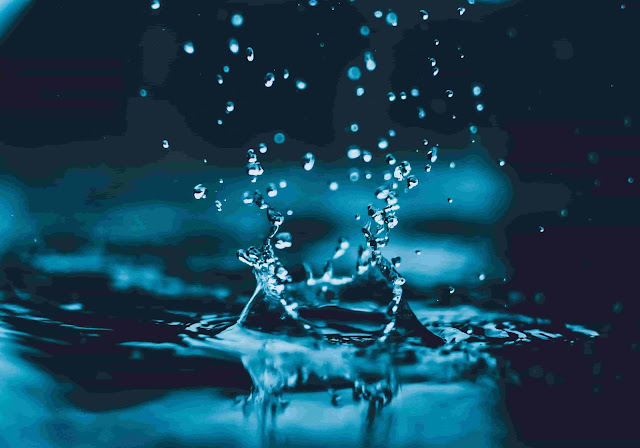# What is ionic product of water?Ionic Product of Water

Ionic product of water is defined as the product of molar concentrations of H+ and OH- at a temperature that is specified. It is the product of concentration those ions obtained by dissociation of water. The ionic product of water is represented by Kw.

Water is made up of a molecule of oxygen and two molecules of hydrogen. Water tends to self ionize and this process is known as autoprotolysis. It splits to form its constituents that are hydrogen and hydroxide ions. But this hydrogen ion is very reactive and tends to form hydronium ion H3O+

But the thing is that hydronium ion is a very strong acid and the hydroxide ion is a very strong base. They quickly form form water again and attain equilibrium.

Thus at any given time, there are a very few hydronium and hydroxide ions that are present.

## What is the effect of temperature on ionic product of water?

The process by which the hydronium and hydroxide ions form water is an endothermic process and thus the reaction absorbs heat. According to Le Chatelier Principle, if the temperature of water is increased, the equilibrium would move to lower the temperature. This is achieved by absorbing the heat.

When there is a rise in temperature, there also is an increase in the concentrations of H+ and OH- and thus the values of the ionic product also increases. That is why it is said that the ionic product of water is temperature dependent.

${K}_{\text{w}}=\left[{\text{H}}^{+}\right]\left[{\text{OH}}^{-}\right]=1.0×{10}^{-14}$

${}^{}$
The value of Kw varies with temperature. It is usually 1.00 x 10-14 mol2 dm-6 at room temperature.

The value of H+ and OH- is therefore:

$\left[{\text{H}}^{+}\right]=\left[{\text{OH}}^{-}\right]=1.0×{10}^{-7}$

We calculated the molarity of each ion by taking the square root of Kw.

The following table shows how the values of Kw varies with temperature. The pH of water also is affected.

T (°C) Kw (mol2 dm-6) pH
0 0.114 x 10-14 7.47
10 0.293 x 10-14 7.27
20 0.681 x 10-14 7.08
25 1.008 x 10-14 7.00
30 1.471 x 10-14 6.92
40 2.916 x 10-14 6.77
50 5.476 x 10-14 6.63
100 51.3 x 10-14 6.14

Table credit: Chemguide

$\phantom{\rule{0ex}{0ex}}\mathrm{K}=\frac{\left[{\mathrm{H}}^{+}\right]\left[{\mathrm{OH}}^{-}\right]}{\left[{\mathrm{H}}_{2}\mathrm{O}\right]}$

$\phantom{\rule{0ex}{0ex}}\mathrm{K}\left[{\mathrm{H}}_{2}\mathrm{O}\right]=\left[{\mathrm{H}}^{+}\right]\left[{\mathrm{OH}}^{-}\right]$

K w = [ H + ] [ OH  ]

## Significance of Ionic product of water

The ionic product of water is very essential in calculations. It helps us to calculate the acid-base balance related questions. It finds its use in human physiology as well, where it again helps with the acid-base balance in the human body for proper functioning.

## Determination of ionic product of water

Let us take and example of hydrochloric acid. It is known that it is a strong acid and therefore it would completely ionize in solution. The following example would show how to calculate the H+ and the OH- in a solution of $2.0×{10}^{-3}\phantom{\rule{mediummathspace}{0ex}}$

We know that $\left[\text{HCl}\right]=2.0×{10}^{-3}\phantom{\rule{mediummathspace}{0ex}}$

$\begin{array}{rl}\left[{\text{H}}^{+}\right]& =2.0×{10}^{-3}\phantom{\rule{mediummathspace}{0ex}}\\ {K}_{\text{w}}& =\left[{\text{H}}^{+}\right]\left[{\text{OH}}^{-}\right]=1.0×{10}^{-14}\\ \left[{\text{OH}}^{-}\right]& =\frac{{K}_{\text{w}}}{\left[{\text{H}}^{+}\right]}=\frac{1.0×{10}^{-14}}{2.0×{10}^{-3}}=5.0×{10}^{-12}\phantom{\rule{mediummathspace}{0ex}}\end{array}$

As the H+ is higher than OH-, it means that the solution is acidic. If the value of OH- would have been greater then we would've concluded the solution to be basic.